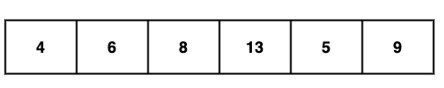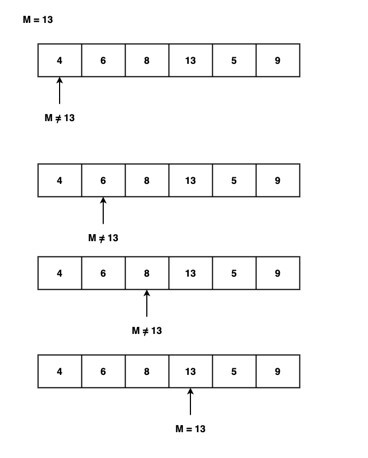# Swift Program to Implement Linear Search Algorithm

In Swift, the Linear search algorithm is the easiest search algorithm. In a linear search algorithm, searching starts from index 0 and checks all the elements of the given array/sequence one by one until the desired element is found. If the desired element is found, then return the index of that element. If the desired element is not found, then return null.

For example

We have an element M = 13, now we search M in the following array using a linear search algorithm −The linear search algorithm starts from index 0 and compares M=13 with each element one by one as shown in the below image −Here, in the above image, the M element is found in the given array so it returns index 3. Otherwise, it will return an element not found.

## Algorithm

• Step 1 − Create a function that takes two arguments that are an array of elements of generic type ’M’ and a target element of type ‘M’.

• Step 2 − Inside the function, run a for loop which iterates through each element of the array and checks if they are equal to the target element or not.

• Step 3 − If the target element is equal to the current element, then return the index of that element.

• Step 4 − If the target element is not found in the given array, then this function returns nil.

• Step 5 − Outside the function create an array and a target element.

• Step 6 − Now call the function and pass the array and the target element in it to get the result.

• Step 7 − Print the output.

## Example 1

In the following example, we create a function named as linearSearchAlgorithm to implement the linear search algorithm. This function takes two arguments: array = [2, 4, 19, 5, 23, 7, 16, 5, 18] and target element = 23. Now the function runs a for loop starting from index 0 and checks if the current element is equal to the target element = 23 using the == operator. If the current element is equal to the target element then return the index of the current element. Otherwise, move to the next element. This process continues till the end of the array. If the target is not found, then this function returns nil. Here in our example target element is found in the given array. So we get “Element 23 is found at index 4” as an output.

import Foundation
import Glibc

func linearSearchAlgorithm<M: Equatable>(_ array: [M], _ element: M) -> Int? {
for x in 0..<array.count {
if array[x] == element {
return x
}
}
return nil
}

let arr = [2, 4, 19, 5, 23, 7, 16, 5, 18]
let ele = 23
if let indexValue = linearSearchAlgorithm(arr, ele) {
print("Element \(ele) is found at index \(indexValue)")
} else {
}


## Output

Element 23 is found at index 4


## Example 2

In the following example, we will create a function named as linearSearchAlgorithm to implement the linear search algorithm. This function takes two arguments: array = [2, 4, 6, 12, 56, 3, 8] and target element = 6. Now the function iterates through each element of the array with the help of the enumerated() method. The enumerated() method returns a tuple that contains the element’s index and the value in each iteration. So if the target element is matched with the current element, then this function returns the index of that element. Otherwise, return nil. Here in our example target element is found in the given array. So the function return indexValue = 2.

import Foundation
import Glibc

func linearSearchAlgorithm<M: Equatable>(_ array: [M], _ element: M) -> Int? {
for (indexValue, Celement) in array.enumerated() {
if Celement == element {
return indexValue
}
}
return nil
}

let arr = [2, 4, 6, 12, 56, 3, 8]
let ele = 6
if let indexValue = linearSearchAlgorithm(arr, ele) {
print("Element \(ele) is found at index \(indexValue)")
} else {
}


## Output

Element 6 is found at index 2


## Conclusion

So this is how we can implement a linear search algorithm. Linear search algorithms can work with both sorted or unsorted sequences. The best execution time of the linear search algorithm is 1 whereas the worst execution time is n, where n represents the total number of elements in the search array. Although the linear search algorithm is the easiest algorithm, it is not suitable for larger arrays.

Updated on: 21-Apr-2023

215 Views